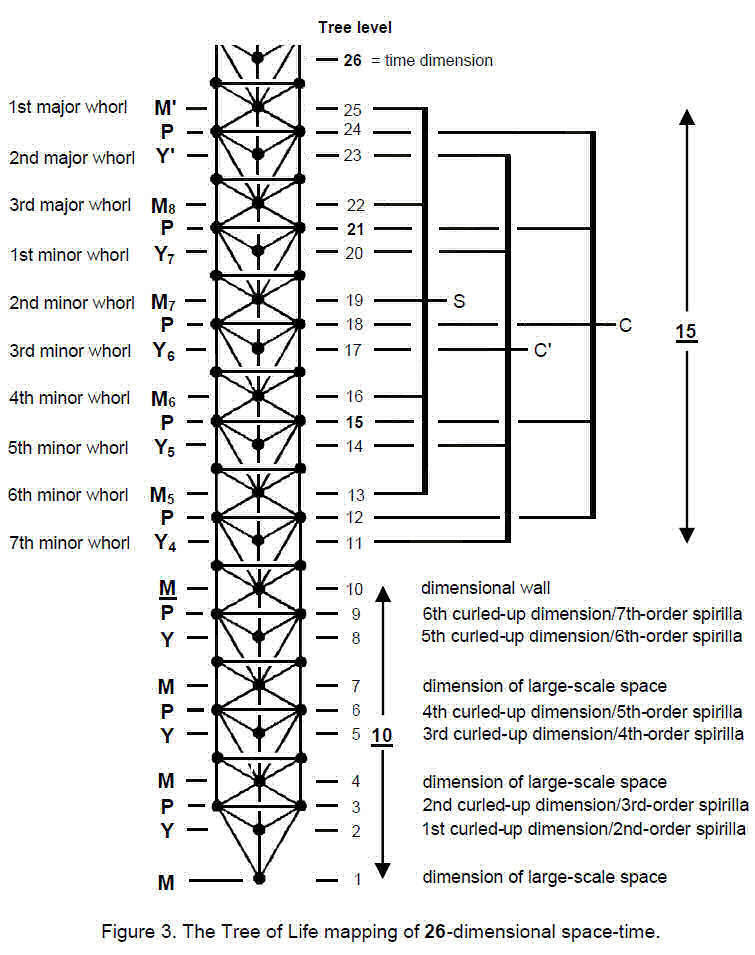top of page
Search

# Multidimensional superstring vibrations

There are infinite numbers so that means there are infinite frequencys

This means there are infinite frequencys a superstring(infinite tetrahedron grid) can have

This means there are infinite dimensions

The superstrings that make up the dimension you are in are 7 dimensions higher(the 3 dimension is made out of superstring vibrating in the 10 dimension )

There are 12 vibrational dimensions with infinite dimensions in between

After the 12 vibrational dimensions there are infinite sub multidimensional levels